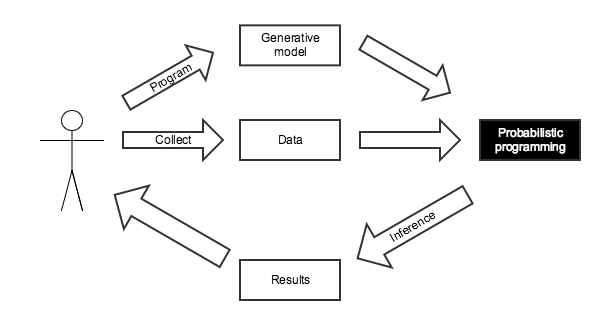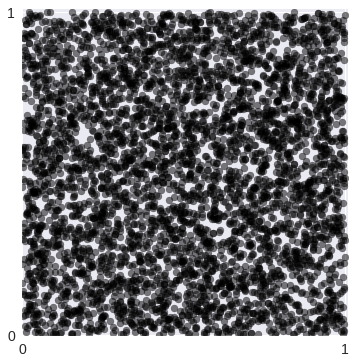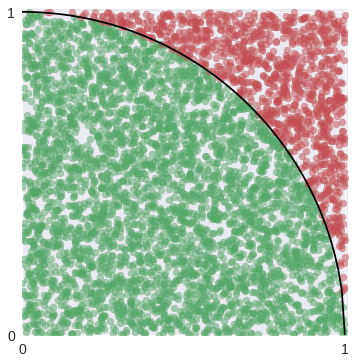A Dockerfile that will produce a container with all the dependencies necessary to run this notebook is available here.

In :
%matplotlib inline

In :
from warnings import filterwarnings

In :
from matplotlib import pyplot as plt
from matplotlib.patches import Ellipse
from matplotlib.ticker import StrMethodFormatter
import numpy as np
import pandas as pd
import scipy as sp
import seaborn as sns
from statsmodels.datasets import get_rdataset

In :
# configure pyplot for readability when rendered as a slideshow and projected
plt.rc('figure', figsize=(8, 6))

LABELSIZE = 14
plt.rc('axes', labelsize=LABELSIZE)
plt.rc('axes', titlesize=LABELSIZE)
plt.rc('figure', titlesize=LABELSIZE)
plt.rc('legend', fontsize=LABELSIZE)
plt.rc('xtick', labelsize=LABELSIZE)
plt.rc('ytick', labelsize=LABELSIZE)

In :
filterwarnings('ignore', 'findfont')

In :
blue, green, red, purple, gold, teal = sns.color_palette()

pct_formatter = StrMethodFormatter('{x:.1%}')

In :
SEED = 54902 # from random.org, for reproducibility

np.random.seed(SEED)


# Open Source Bayesian Inference in Python with PyMC3¶## Who am I?¶## Bayesian Inference¶

A motivating question:

A rare disease is present in one out of one hundred thousand people. A test gives the correct diagnosis 99.9% of the time. What is the probability that a person that tests positive has the disease?

### Conditional Probability¶

Conditional probability is the probability that one event will happen, given that another event has occured.

\begin{align*} P(A\ |\ B) & = \textrm{the probability that } A \textrm{ will happen if we know that } B \textrm{ has happened} \\ & = \frac{P(A \textrm{ and } B)}{P(B)}. \end{align*}

Our question,

A rare disease is present in one out of one hundred thousand people. A test gives the correct diagnosis 99.9% of the time. What is the probability that a person that tests positive has the disease?

becomes

\begin{align*} P(+) & = 10^{-5} \\ \\ P(\textrm{Test } +\ |\ +) & = 0.999 \\ P(\textrm{Test } -\ |\ -) & = 0.999 \\ \\ P(+\ |\ \textrm{Test } +) & =\ \textbf{?} \end{align*}

### Bayes' Theorem¶

Bayes' theorem shows how to get from $P(B\ |\ A)$ to $P(A\ |\ B)$.Bayes' Theorem follows directly from the definition of conditional probability.

\begin{align*} P(A\ |\ B) P(B) & = P(A \textrm{ and } B) \\ & = P(B \textrm{ and } A) \\ & = P(B\ |\ A) P(A). \end{align*}

Dividing by $P(B)$ yields Bayes' theorem. Each component of Bayes' Theorem has a name. The posterior distribution is equal to the joint distribution divided by the marginal distribution of the evidence.

$$\color{red}{P(A\ |\ B)} = \frac{\color{blue}{P(B\ |\ A)\ P(A)}}{\color{green}{P(B)}}.$$

For many useful models the marginal distribution of the evidence is hard or impossible to calculate analytically.

The first step below follows from Bayes' theorem, the second step follows from the law of total probability.

\begin{align*} P(+\ |\ \textrm{Test } +) & = \frac{P(\textrm{Test } +\ |\ +) P(+)}{P(\textrm{Test } +)} \\ & = \frac{P(\textrm{Test } +\ |\ +) P(+)}{P(\textrm{Test } +\ |\ +) P(+) + P(\textrm{Test } +\ |\ -) P(-)} \end{align*}
\begin{align*} P(+) & = 10^{-5} \\ P(\textrm{Test } +\ |\ +) & = 0.999 \\ P(\textrm{Test } -\ |\ -) & = 0.999 \\ \\ P(+\ |\ \textrm{Test } +) & = \frac{P(\textrm{Test } +\ |\ +) P(+)}{P(\textrm{Test } +\ |\ +) P(+) + P(\textrm{Test } +\ |\ -) P(-)} \\ & = \frac{0.999 \times 10^{-5}}{0.999 \times 10^{-5} + 0.001 \times \left(1 - 10^{-5}\right)} \end{align*}

Strikingly, a person that tests positive has a less than 1% chance of actually having the disease! (Worryingly, only 21% of doctors answered this question correctly in a recent study.)

In :
0.999 * 1e-5 / (0.999 * 1e-5 + 0.001 * (1 - 1e-5))

Out:
0.009891284975940117

## Probabilistic Programming for Bayesian Inference¶This calculation was tedious to do by hand, and only had a closed form answer because of the simplicity of this situation.

We know the disease is present in one in one hundred thousand people.

The Bernoulli distribution gives the probability that a weighted coin flip comes up heads. If $X \sim \textrm{Bernoulli}(p),$

\begin{align*} P(X = 1) & = p \\ P(X = 0) & = 1 - p. \end{align*}
In :
import pymc3 as pm

with pm.Model() as disease_model:
has_disease = pm.Bernoulli('has_disease', 1e-5)


If a person has the disease, there is a 99.9% chance they test positive. If they do not have the disease, there is a 0.1% chance they test positive.

In :
with disease_model:
p_test_pos = has_disease * 0.999 + (1 - has_disease) * 0.001


This person has tested positive for the disease.

In :
with disease_model:
test_pos = pm.Bernoulli('test_pos', p_test_pos, observed=1)


What is the probability that this person has the disease?

In :
with disease_model:
disease_trace = pm.sample(draws=10000, random_seed=SEED)

Assigned BinaryGibbsMetropolis to has_disease
100%|██████████| 10500/10500 [00:04<00:00, 2427.46it/s]


The disease_trace object contains samples from the posterior distribution of has_disease, given that we have observed a positive test.

In :
disease_trace['has_disease']

Out:
array([0, 0, 0, ..., 0, 0, 0])

The mean of the posterior samples of has_disease is the posterior probability that the person has the disease, given that they tested positive.

In :
disease_trace['has_disease'].mean()

Out:
0.0104
In :
0.999 * 1e-5 / (0.999 * 1e-5 + 0.001 * (1 - 1e-5))

Out:
0.009891284975940117

### Monte Carlo Methods¶

Monte Carlo methods use random sampling to approximate quantities that are difficult or impossible to calculate analytically. They are used extensively in Bayesian inference, where the marginal distribution of the evidence is usually impossible to calculate analytically for interesting models.

First we generate 5,000 points randomly distributed in a square of area one.

In :
N = 5000

x, y = np.random.uniform(0, 1, size=(2, N))

In :
fig, ax = plt.subplots()
ax.set_aspect('equal');

ax.scatter(x, y, c='k', alpha=0.5);

ax.set_xticks([0, 1]);
ax.set_xlim(0, 1.01);

ax.set_yticks([0, 1]);
ax.set_ylim(0, 1.01);In :
fig

Out:By counting the number of these points that fall inside the quarter circle of radius one, we get an approximation of the area of this quarter circle, which is $\frac{\pi}{4}$.

In :
in_circle = x**2 + y**2 <= 1

In :
fig, ax = plt.subplots()
ax.set_aspect('equal');

x_plot = np.linspace(0, 1, 100)
ax.plot(x_plot, np.sqrt(1 - x_plot**2), c='k');

ax.scatter(x[in_circle], y[in_circle], c=green, alpha=0.5);
ax.scatter(x[~in_circle], y[~in_circle], c=red, alpha=0.5);

ax.set_xticks([0, 1]);
ax.set_xlim(0, 1.01);

ax.set_yticks([0, 1]);
ax.set_ylim(0, 1.01);In :
fig

Out: